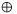A better shot; use:

st, u     read as `if s then t else u'

Convert to If-then-else Normal Form (INF).

What is INF?

What is the INF for the following:

xyz

We can draw INFs as binary decision trees. What is a decision tree? What is the decision tree for this formula?

Decision trees are still not acceptable: why not?